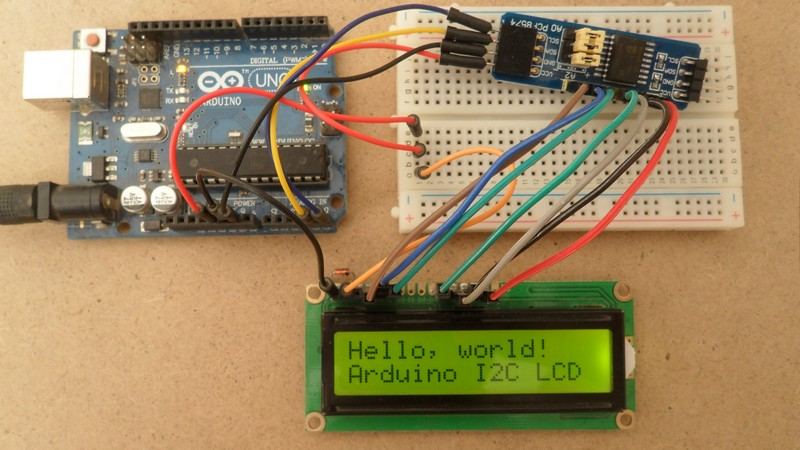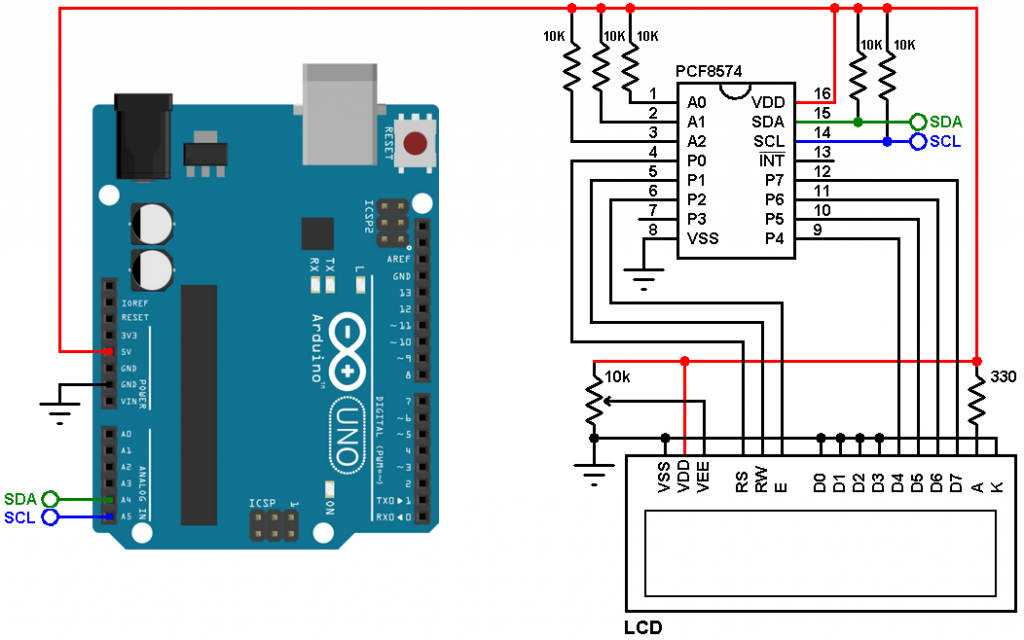# Interfacing Arduino with I2C LCD

Generally to be able to use an LCD display we need at least 6 free pins, but the number of pins can be minimized with the help of external components like PCF8574 (or PCF8574A) I2C I/O expander, that’s allows us to use only 2 pins from our microcontroller. This small post shows how to connect the Arduino with I2C LCD provided with PCF8574 I/O expander.

Hardware Required:

• Arduino board
• LCD screen (16×2, 20×4 …)
• PCF8574 I/O expander (or PCF8574A)   —   PCF8574 datasheet
• 5 x 10k ohm resistor
• 330 ohm resistor
• 10k ohm variable resistor or potentiometer
• Jumper wiresInterfacing Arduino with I2C LCD circuit:
Example circuit diagram is shown in the image below.(All grounded terminals are connected together)

The main component of the I2C LCD display is the PCF8574 I/O expander, with only two pins SDA and SCL we get a maximum of 8 pins from P0 to P7. PCF8574A also can be used but it has a different address.

All LCD data pins are connected to the PCF8574 where: RS, RW, E, D4, D5, D6 and D7 are connected to P0, P1, P2, P4, P5, P6 and P7 respectively.

PCF8574 I/O expander SDA and SCL pins are connected to Arduino pin A4 and A5 respectively (Arduino SDA and SCL pins).

PCF8574 I/O expander A0, A1 and A2 pins are the address pins which decide the I2C address of the chip. In this example each pin is connected to +5V through a 10k ohm resistor (the 10k resistor is optional, each pin can be connected directly to +5V).

The I2C address of the PCF8574 is: 0x20 | A2 A1 A0 ( | means OR)
In our circuit A2, A1 and A0 are connected to +5V (through 10k resistors) which means the I2C address is equal to 0x20 | 7 = 0x27

If the PCF8574A is used instead of the PCF8574 the I2C address is: 0x38 | 7 = 0x3F.

Interfacing Arduino with I2C LCD code:
In this interfacing I used a small library named LiquidCrystal_I2C (LiquidCrystal_I2C.h), this library simplifies the Arduino code, it can be downloaded from the links below. After downloading the library, unzip the folder and add it to Arduino libraries folder (for example: C:\Program Files\Arduino\libraries):